# Статьи журнала - Владикавказский математический журнал

Все статьи: 834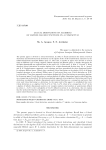2-local derivations on algebras of matrix-valued functions on a compactum

Ayupov Shavkat Abdullayevich, Arzikulov Farhodjon Nematjonovich

Статья научная

The present paper is devoted to 2-local derivations. In 1997, P. Semrl introduced the notion of 2-local derivations and described 2-local derivations on the algebra B(H) of all bounded linear operators on the infinite-dimensional separable Hilbert space H. After this, a number of paper were devoted to 2-local maps on different types of rings, algebras, Banach algebras and Banach spaces. A similar description for the finite-dimensional case appeared later in the paper of S. O. Kim and J. S. Kim. Y. Lin and T. Wong described 2-local derivations on matrix algebras over a finite-dimensional division ring. Sh. A. Ayupov and K. K. Kudaybergenov suggested a new technique and have generalized the above mentioned results for arbitrary Hilbert spaces. Namely they considered 2-local derivations on the algebra B(H) of all linear bounded operators on an arbitrary Hilbert space H and proved that every 2-local derivation on B(H) is a derivation. Then there appeared several papers dealing with 2-local derivations on associative algebras. In the present paper 2-lo\-cal derivations on various algebras of infinite dimensional matrix-valued functions on a compactum are described. We develop an algebraic approach to investigation of derivations and \mbox{2-local} derivations on algebras of infinite dimensional matrix-valued functions on a compactum and prove that every such 2-local derivation is a derivation. As the main result of the paper it is established that every \mbox{2-local} derivation on a ∗-algebra C(Q,Mn(F)) or C(Q,Nn(F)), where Q is a compactum, Mn(F) is the ∗-algebra of infinite dimensional matrices over complex numbers (real numbers or quaternoins) defined in section 1, Nn(F) is the ∗-subalgebra of Mn(F) defined in section 2, is a derivation. Also we explain that the method developed in the paper can be applied to Jordan and Lie algebras of infinite dimensional matrix-valued functions on a compactum.

Бесплатно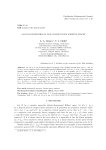2-local isometries of non-commutative Lorentz spaces

Alimov Akrom A., Chilin Vladimir I.

Статья научная

Let M be a von Neumann algebra equipped with a faithful normal finite trace τ, and let S(M,τ) be an ∗-algebra of all τ-measurable operators affiliated with M. For x∈S(M,τ) the generalized singular value function μ(x):t→μ(t;x), t>0, is defined by the equality μ(t;x)=inf{∥xp∥M:p2=p∗=p∈M,τ(1-p)≤t}. Let ψ be an increasing concave continuous function on [0,∞) with ψ(0)=0, ψ(∞)=∞, and let Λψ(M,τ)={x∈S(M,τ): ∥x∥ψ=∫∞0μ(t;x)dψ(t)

Бесплатно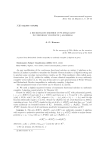A Beckenbach - Dresher type inequality in uniformly complete f-algebras

Kusraev Anatoly G.

Статья научная

The Beckenbah-Dresher type inequality in uniformly complete f-algebras

Бесплатно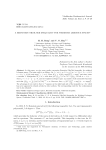A Bernstein-Nikol'skii inequality for weighted Lebesgue spaces

Bang Ha Huy, Huy Vu Nhat

Статья научная

In this paper, we give some results concerning Bernstein-Nikol'skii inequality for weighted Lebesgue spaces. The advantage of our result is that m-ϱ appears on the right hand side of the inequality (ϱ>0), which has never appeared in related articles by other authors. The corresponding result for the n-dimensional case is also obtained.

Бесплатно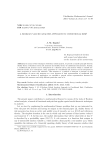A Boolean valued analysis approach to conditional risk

Zapata Jose Miguel

Статья научная

By means of the techniques of Boolean valued analysis, we provide a transfer principle between duality theory of classical convex risk measures and duality theory of conditional risk measures. Namely, a conditional risk measure can be interpreted as a classical convex risk measure within a suitable set-theoretic model. As a consequence, many properties of a conditional risk measure can be interpreted as basic properties of convex risk measures. This amounts to a method to interpret a theorem of~dual representation of convex risk measures as a new theorem of dual representation of conditional risk measures. As an instance of application, we establish a general robust representation theorem for conditional risk measures and study different particular cases of it.

Бесплатно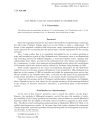A by-proxy talk on Alexandrov's contribution

Статья научная

This talk presents the unpublished articles by O. A. Ladyzhenskaya, Yu. G. Reshetnyak, and V. A. Zalgaller concerning the nomination of A. D. Alexandrov for the Wolf Prize in 1995.

Бесплатно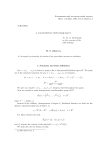A caccioppoli type inequality

Alborova Mira Soslanovna

Статья научная

A Caccioppoli type inequality for solutions of the quasi-elliptic equations is established.

Бесплатно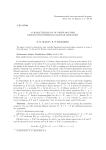A characterization of order bounded disjointness preserving bilinear operators

Kusraev Anatoly Georgievich, Kutateladze Semen Samsonovich

Статья научная

The paper is aimed to characterize order bounded disjointness preserving bilinear operators in terms of their -spaces. To this end the Boolean valued analysis approach is employed.

Бесплатно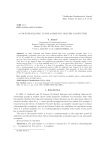A counter-example to the Andreoti-Grauert conjecture

Alaoui Youssef

Статья научная

In 1962, Andreotti and Grauert showed that every q-complete complex space X is cohomologically q-complete, that is for every coherent analytic sheaf F on X, the cohomology group Hp(X,F) vanishes if p≥q. Since then the question whether the reciprocal statements of these theorems are true have been subject to extensive studies, where more specific assumptions have been added. Until now it is not known if these two conditions are equivalent. Using test cohomology classes, it was shown however that if X is a Stein manifold and, if D⊂X is an open subset which has C2 boundary such that Hp(D,OD)=0 for all p≥q, then D is q-complete. The aim of the present article is to give a counterexample to the conjecture posed in 1962 by Andreotti and Grauert  to show that a cohomologically q-complete space is not necessarily q-complete. More precisely, we show that there exist for each n≥3 open subsets Ω⊂Cn such that for every F∈coh(Ω), the cohomology groups Hp(Ω,F) vanish for all p≥n-1 but Ω is not (n-1)-complete.

Бесплатно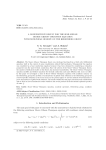A nonexistence result for the semi-linear Moore-Gibson-Thompson equation with nonlinear memory on the Heisenberg group

Georgiev Svetlin G., Hakem Ali

Статья научная

The Moore-Gibson-Thompson theory was developed starting from a third order differential equation, built in the context of some consideration related fluid mechanics. Subsequently the equation was considered as a heat conduction equation because it has been obtained by considering a relaxation parameter into the type III heat conduction. Since the advent of the Moore-Gibson-Thompson theory, the number of dedicated studies to this theory has increased considerably. The Moore-Gibson-Thompson equation modifies and defines equations for thermal conduction and mass diffusion that occur in solids. In this paper we investigate a class of Moore-Gibson-Thompson equation with nonlinear memory on the Heisenberg group.The problem of nonexistence of global weak solutions in the Heisenberg group has received specific attention in the recent years. In the present paper we use the method of test functions to prove nonexistence of global weak solutions. The results obtained in this paper extend several contributions and we focus on new nonexistence results which are due to the presence of the fractional Laplacian operator of order σ/2.

Бесплатно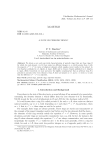A note on periodic rings

Danchev Peter V.

Статья научная

We obtain a new and non-trivial characterization of periodic rings (that are those rings R for which, for each element x in R, there exists two different integers m, n strictly greater than 1 with the property xm=xn) in terms of nilpotent elements which supplies recent results in this subject by Cui-Danchev published in (J. Algebra & Appl., 2020) and by Abyzov--Tapkin published in (J. Algebra & Appl., 2022). Concretely, we state and prove the slightly surprising fact that an arbitrary ring R is periodic if, and only if, for every element x from R, there are integers m>1 and n>1 with m≠n such that the difference xm-xn is a nilpotent.

Бесплатно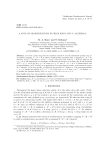A note on semiderivations in prime rings and C*-algebras

Raza Mohd Arif, Rehman Nadeem Ur

Статья научная

Let R be a prime ring with the extended centroid C and the Matrindale quotient ring Q. An additive mapping F:R→R is called a semiderivation associated with a mapping G:R→R, whenever F(xy)=F(x)G(y)+xF(y)=F(x)y+G(x)F(y) and F(G(x))=G(F(x)) holds for all x,y∈R. In this manuscript, we investigate and describe the structure of a prime ring R which satisfies F(xm∘yn)∈Z(R) for all x,y∈R, where m,n∈Z+ and F:R→R is a semiderivation with an automorphism ξ of R. Further, as an application of our ring theoretic results, we discussed the nature of C∗-algebras. To be more specific, we obtain for any primitive C∗-algebra A. If an anti-automorphism ζ:A→A satisfies the relation (xn)ζ+xn∗∈Z(A) for every x,y∈A, then A is C∗-W4-algebra, i.e., A satisfies the standard identity W4(a1,a2,a3,a4)=0 for all a1,a2,a3,a4∈A.

Бесплатно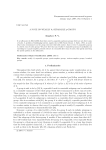A note on weakly \Aleph_1-separable P-groups

Danchev peteR. V.

Статья научная

It is well-known by Hill-Griffith that there exist \aleph_1-separable \$p\$-primary groups which are not direct sums of cycles. A problem of challenging interest, mainly due to Hill (Rocky Mount. J. Math., 1971), is under what extra circumstances on the group structure this holds untrue, that is every \aleph_1-separable p-group is a direct sum of cyclic groups. We prove here that any weakly \aleph_1-separable p-group of cardinality not exceeding \aleph_1 is quasi-complete precisely when it is a bounded direct sum of cycles, thus partly answering the posed question in the affirmative.

Бесплатно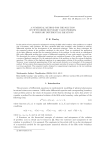A numerical method for the solution of fifth order boundary value problem in ordinary differential equations

Pandey Pramod Kumar

Статья научная

In this article we have proposed a technique for solving the fifth order boundary value problem as a coupled pair of boundary value problems. We have considered fifth order boundary value problem in ordinary differential equation for the development of the numerical technique. There are many techniques for the numerical solution of the problem considered in this article. Thus we considered the application of the finite difference method for the numerical solution of the problem. In this article we transformed fifth order differential problem into system of differential equations of lower order namely one and four. We discretized the system of differential equations into considered domain of the problem. Thus we got a system of algebraic equations. For the numerical solution of the problem, we have the system of algebraic equations. The solution of the algebraic equations is an approximate solution of the problem considered. Moreover we get numerical approximation of first and second derivative as a byproduct of the proposed method. We have shown that proposed method is convergent and order of accuracy of the proposed method is at lease quadratic. The numerical results obtained in computational experiment on the test problems approve the efficiency and accuracy of the method.

Бесплатно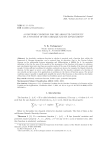A pointwise condition for the absolute continuity of a function of one variable and its applications

Vodopyanov Sergey K.

Статья научная

An absolutely continuous function in calculus is precisely such a function that, within the framework of Lebesgue integration, can be restored from its derivative, that is, the Newton-Leibniz theorem on the relationship between integration and differentiation is fulfilled for it. An equivalent definition is that the the sum of the moduli of the increments of the function with respect to arbitrary pair-wise disjoint intervals is less than any positive number if the sum of the lengths of the intervals is small enough. Certain sufficient conditions for absolute continuity are known, for example, the Banach-Zaretsky theorem. In this paper we prove a new sufficient condition for the absolute continuity of a function of one variable and give some of its applications to problems in the theory of function spaces. The proved condition makes it possible to significantly simplify the proof of the theorems on the pointwise description of functions of the Sobolev classes defined on Euclidean spaces and Сarnot groups.

Бесплатно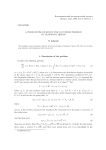A priori estimate result for an inverse problem of transport theory

Lahrech Samir

Статья научная

We establish a priori estimate result for an inverse problem of transport theory. We refer to , where some existence and uniqueness results are proved.

Бесплатно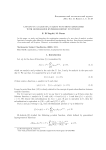A study on a class of \$p\$-valent functions associated with generalized hypergeometric functions

El-Yagubi Entisar, Darus Maslina

Статья научная

In this paper, we study and introduce the majorization properties of a new class of analytic \$p\$-valent functions of complex order defined by the generalized hypergeometric function. Some known consequences of our main result will be given. Moreover, we investigate the coefficient estimates for this class.

Бесплатно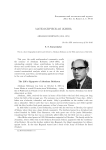Abraham Robinson (1918-1974)

Персоналии

This is a short biographical sketch and tribute to Abraham Robinson on the 95th anniversary of his birth.

Бесплатно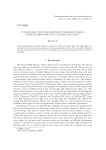An EOQ model with time-dependent increasing demand under JIT philosophy for a distributor/agent

Sana Shib Sankar

Статья научная

This article generalizes an EOQ model for a distributor/agent with Just-in-Time (JIT) philosophy. It is assumed that the demand of seasonal goods is an increasing function of time. The optimality condition of the associated objective function is derived analytically. Also, the model is illustrated with the numerical examples.

Бесплатно

Журнал